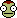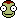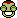# Who will solve this first ?

## Recommended Posts

I guess I'm nitpicking here.

The question "What height does the water reach" should be "what maximum height can the water reach".

If that is the actual question then there is no point in mentioning the 500l/s

If I force 500l/s through an infinitely small hole i theoretically get infinite speed. If I don't have infinite power, I obviously can not do that (the power at hand is stated).

So what is the 500l/s mention for? is that a distraction?

##### Share on other sites
I guess I'm nitpicking here.

The question "What height does the water reach" should be "what maximum height can the water reach".

If that is the actual question then there is no point in mentioning the 500l/s

If I force 500l/s through an infinitely small hole i theoretically get infinite speed. If I don't have infinite power, I obviously can not do that (the power at hand is stated).

So what is the 500l/s mention for? is that a distraction?

The pump has a power that it supplies to the water. Obviously the speed that the water is accelerate to with this given power depends on how many litres the power is supplied to.

##### Share on other sites
The pump has a power that it supplies to the water. Obviously the speed that the water is accelerate to with this given power depends on how many litres the power is supplied to.

No.

(charlimit makes me post this, too.)

Edited by Kerbin Dallas Multipass
##### Share on other sites
No.

I'm not sure you understand power or maybe just rates in general. Given rate of flow and power, you know final velocity. Both of the former need to be established to deduce the later.

##### Share on other sites
I guess I'm nitpicking here.

The question "What height does the water reach" should be "what maximum height can the water reach".

If that is the actual question then there is no point in mentioning the 500l/s

If I force 500l/s through an infinitely small hole i theoretically get infinite speed. If I don't have infinite power, I obviously can not do that (the power at hand is stated).

So what is the 500l/s mention for? is that a distraction?

Guys, how about I just show him every single step, because I don't think he quite gets what the units have to do with it.

500 L/s

10^6 W

Watch me do magic here.

1000000 W = 1000000 J/s

1000000 J/s / 500 L/s

Okay, take the numbers: 2000

Now take the units: J/s / L/s. Let's simplify. J/s * s/L = J/L

2000 J/L. That's how much energy that is delivered to each liter. This is because the amount of work depends solely on how much water is going through at once, and how much power is supplied, as that determines how much work is supplied to each liter. Notably, this ratio is determined by the size of the hole through which the water exits, however, knowing how big the hole is is not required if you already know the throughput and the power (because then you know their ratio already, and the only thing that matters is that ratio).

When you play with infinities, things break down because you hit a mathematically singularity here, and things don't work right at all then. Just know that larger holes require less power to force the same throughput as smaller holes.

Moving on,

1 Liter of water is also 1 kg of water, due to its density.

So, 2000 J/kg.

dU = m * g * h. Or dU/m = g * h

Assume 1 kg of water, it doesn't matter, because it instantly simplifies out. 2000J/kg * 1 kg/1 kg = 9.81 * h.

h = 203.87.

##### Share on other sites

Well explained, Kohani.

##### Share on other sites

Its pretty fun to realize that my fairly easy problem created a big discussion ! (i can give you a harder (better also) one if some of you are interested)

I don't get why some people think that the flow means nothing and cannot be used... Well, kohai khaos explained it very well.

btw, you might have realized that this fountain is not the common foutain you find around in town200m high and 500l/s flow, thats a lot !

##### Share on other sites

Some fire-fighting boats should be capable of something comparable.

And sure, why not? We might even just make this into physics brain-teasers thread if it goes well. I have a few tricky ones.##### Share on other sites

Why the hell did I not get taught this stuff in school?! Seriously! I live in Britain and when I was a student (only 9 years ago(I'm 25)) we never got taught anything even resembling this sort of stuff. Now I know why I never stayed in edumacation. I have learnt more useful every day stuff outside of school. Maybe that's my curious nature or simply the fact we get taught the answers to the questions for the exams in British schools?!

Thankyou for giving me another lessonTweety

##### Share on other sites
I guess I'm nitpicking here.

The question "What height does the water reach" should be "what maximum height can the water reach".

No.

You're rewriting the question. If you allow us to apply all that energy to an arbitrary mass of water, we can get any answer you like. Instead, the question specifies a mass by giving us the ratio between water flow and power.

##### Share on other sites
Why the hell did I not get taught this stuff in school?! Seriously! I live in Britain and when I was a student (only 9 years ago(I'm 25)) we never got taught anything even resembling this sort of stuff. Now I know why I never stayed in edumacation. I have learnt more useful every day stuff outside of school. Maybe that's my curious nature or simply the fact we get taught the answers to the questions for the exams in British schools?!

Thankyou for giving me another lessonTweety

This is (and has been since GCSE was introduced) taught at physics GCSE.

##### Share on other sites

Okay, here is the next problem : (harder) max time allowed to resolve it : 20 minutes !

the guys who love calculations about work and energy will be happyA 1600kg car is on a 6Ã‚Â° inclinated slope. The car is old and rusty : the force of friction due to its movement will always be 800N (whatever its speed, its to make things simpler). The car is abandonned and has no initial velocity. After what distance on the slope does the car reach 36km/h ?

EDIT : the first to solve it gets some rep

Edited by Hcube
##### Share on other sites
Okay, here is the next problem : (harder) max time allowed to resolve it : 20 minutes !

the guys who love calculations about work and energy will be happyA 1600kg car is on a 6Ã‚Â° inclinated slope. The car is old and rusty : the force of friction due to its movement will always be 800N (whatever its speed, its to make things simpler). The car is abandonned and has no initial velocity. After what distance on the slope does the car reach 36km/h ?

EDIT : the first to solve it gets some rep

(VÃ‚Â²*m*sin(O))/2*(mg-sin(O)Fr)

Where O is the angle of the slope, m the mass of the car, v the final velocity, g gravitational acceleration and Fr friction

Edited by BlueCosmology
typo
##### Share on other sites
(VÃ‚Â²*m*sin(O))/(mg-Fr)

Where O is the angle of the slope, m the mass of the car, v the final velocity, g gravitational acceleration and Fr friction

Yeah, but just give the plain answer in m so that others can solve it without seeing the solution immediately##### Share on other sites
Yeah, but just give the plain answer in m so that others can solve it without seeing the solution immediatelyEh, plugging numbers into a formula is neither physics nor interesting enough to be bothered doing.

##### Share on other sites

well no rep for you my friendthe calculation is math actually, and it's another interesting thing(although i must agree that right there the calculation is easy and boring)

##### Share on other sites
well no rep for you my friendthe calculation is math actually, and it's another interesting thing(although i must agree that right there the calculation is easy and boring)

Hahah, arithmetic is about as far from maths as possible (you're never going to find a maths paper that is just solving equations for particular values), and it certainly isn't interesting.

I guess my turn to ask a question?

Given a star that's core temperature is atleast an order of magnitude too low for thermal protons to classically fuse, what is the rate of fusion?

Edited by BlueCosmology
##### Share on other sites

Problems that require precise evaluation of sin 6deg for an answer are not fun.

Nor the ones that are ill posed. There is no classical cutoff for fusion, due to Boltzman. And what do you mean by "at least," when answer is so sensitive to available energy.

##### Share on other sites
Problems that require precise evaluation of sin 6deg for an answer are not fun.

Nor the ones that are ill posed. There is no classical cutoff for fusion, due to Boltzman. And what do you mean by "at least," when answer is so sensitive to available energy.

That is not ill posed.

A thermal particle is one that's energy is within a standard deviation of the energy of a particle with mean energy.

By "at least" I mean, at least. The answer is very sensitive to available energy yes.

Edited by BlueCosmology
##### Share on other sites
That is not ill posed.

A thermal particle is one that's energy is within a standard deviation of the energy of a particle with mean energy.

By "at least" I mean, at least. The answer is very sensitive to available energy yes.

What's the pressure, though?

##### Share on other sites
What's the pressure, though?

Call it whatever you'd like? I'd suggest P.

##### Share on other sites
Guys, how about I just show him every single step, because I don't think he quite gets what the units have to do with it.

500 L/s

10^6 W

Watch me do magic here.

1000000 W = 1000000 J/s

1000000 J/s / 500 L/s

Okay, take the numbers: 2000

Now take the units: J/s / L/s. Let's simplify. J/s * s/L = J/L

2000 J/L. That's how much energy that is delivered to each liter. This is because the amount of work depends solely on how much water is going through at once, and how much power is supplied, as that determines how much work is supplied to each liter. Notably, this ratio is determined by the size of the hole through which the water exits, however, knowing how big the hole is is not required if you already know the throughput and the power (because then you know their ratio already, and the only thing that matters is that ratio).

When you play with infinities, things break down because you hit a mathematically singularity here, and things don't work right at all then. Just know that larger holes require less power to force the same throughput as smaller holes.

Moving on,

1 Liter of water is also 1 kg of water, due to its density.

So, 2000 J/kg.

dU = m * g * h. Or dU/m = g * h

Assume 1 kg of water, it doesn't matter, because it instantly simplifies out. 2000J/kg * 1 kg/1 kg = 9.81 * h.

h = 203.87.

Thank you for explaining that. It makes sense. And it answers why both, the flow rate and the power are described in the task.

But is it realistic? As I illustrated above, pumping 500l/s through a 1mÃ‚Â² pipe will not get us anywhere near a 200m fountain, independent of the power at hand. I think that confused me.

If I were to just write down "Since we're pumping water, it depends on the nozzle"... would that be wrong? (I see it's not the desired answer, but isn't that true?)

## Join the conversation

You can post now and register later. If you have an account, sign in now to post with your account.
Note: Your post will require moderator approval before it will be visible.×   Pasted as rich text.   Paste as plain text instead

Only 75 emoji are allowed.